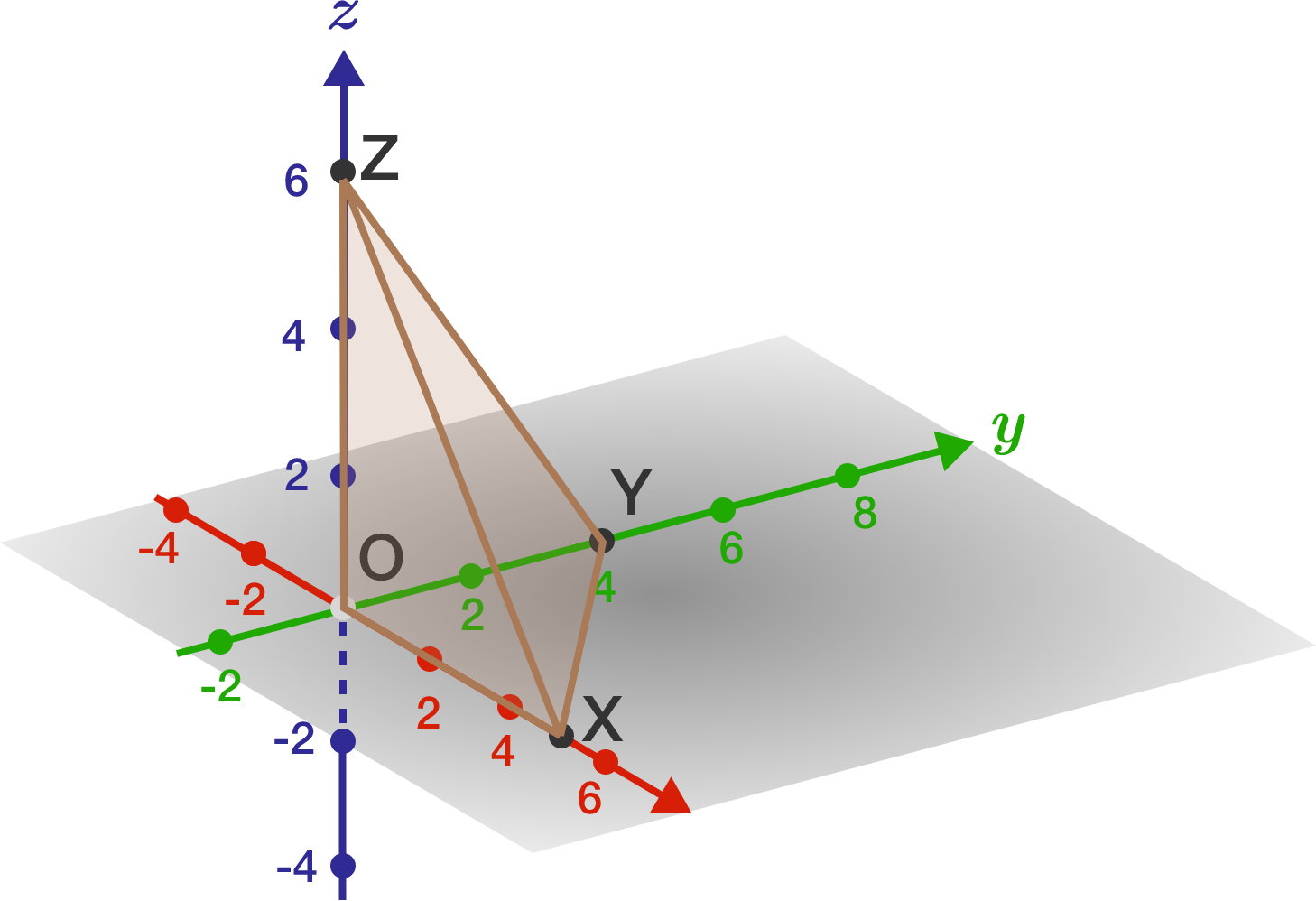# Triangles in 3D!

$O,X,Y,Z$ are four points on a 3D Cartesian space, where $O$ is the origin, $X$ a point on the $x$-axis, $Y$ a point on the $y$-axis, and $Z$ a point on the $z$-axis.

Now, the area of $\triangle OXY$ is given by $a$, the area of $\triangle OYZ$ by $b$, and the area of $\triangle OXZ$ by $c$.

Find the area of $\triangle XYZ$ in terms of $a,b,c$.×